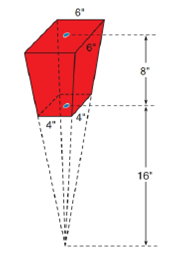Chapter 9.2, Problem 36EElementary Geometry For College St...

7th Edition
Alexander + 2 others
ISBN: 9781337614085

Solutions

Chapter
SectionElementary Geometry For College St...

7th Edition
Alexander + 2 others
ISBN: 9781337614085
Textbook Problem

A popcorn container at a movie theatre has the shape of a frustum of a pyramid. With dimensions as indicated, find the volume (capacity) of the container.To determine

To find:

The volume (capacity) of the container.

Explanation

Given:

The popcorn container at a movie theatre is in the shape of a frustum of a pyramid as shown below

Properties Used:

A pyramid is made by connecting a base to an apex. The base is flat with straight edges, no curves, hence, a polygon and all other faces are triangles.

A regular pyramid is a pyramid whose base is a regular polygon and whose lateral edges are all congruent.

A frustum of a pyramid is the portion of a pyramid that lies between its base and a parallel plane that intersects the pyramid.

The volume V of a pyramid having a base area B and an altitude of length h is given by V=13Bh.

According to the Pythagorean theorem, in a right-angled triangle

hypotenuse2=base2+perpendicular2.

In a regular pyramid, the lengths of the apothem a of the base, the altitude h, and the slant height l satisfy the Pythagorean Theorem; that is, l2=a2+h2.

Approach Used:

i) Calculate the volume of the bigger pyramid VB.

ii) Calculate the volume of the smaller pyramid VS.

iii) Subtract the two to get the volume of the frustum VF=VBVS.

Calculation:

Calculating the volume of the bigger pyramid

with base as a square of side x=6in and height as h=24in.

The area of the square base is

B=x2=62B=36in2

Still sussing out bartleby?

Check out a sample textbook solution.

See a sample solution

The Solution to Your Study Problems

Bartleby provides explanations to thousands of textbook problems written by our experts, many with advanced degrees!

Get Started

Find more solutions based on key concepts